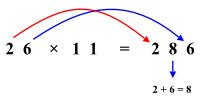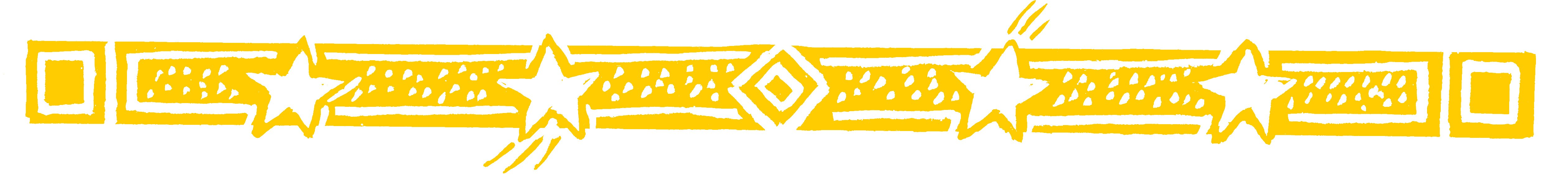Multiplying a number by 11 is incredibly easy -
astonish your parents and friends with this.

To multiply any 2-figure number by 11 we just put
the total of the two figures between the 2 figures.

• 26 x 11 = 286Notice that the outer figures in 286 are the 26
being multiplied.

And the middle figure is just 2 and 6 added up.

• So 72 x 11 = 792

Question Comment !
 Tutorial Title Question Title No Questions 0 Questions Asked 0 Skipped Questions 0 Correctly Answered 0 Wrongly Answered 0 Total Question Attempts 0 Current Question Attempts 0

Skip Question

Reset Test

Halt Test

 No Questions 0 Questions Asked 0 Skipped Questions 0 Correctly Answered 0 Wrongly Answered 0 Total Question Attempts 0

Time Taken

CloseImpress Your Parents (1)

• 77 x 11 = 847

This involves a carry figure because 7 + 7 = 14
we get 77 x 11 = 7147 = 847.Impress Your Parents (2)

• 234 x 11 = 2574

We put the 2 and the 4 at the ends.
We add the first pair 2 + 3 = 5.
and we add the last pair: 3 + 4 = 7.Impress Your Parents (3)